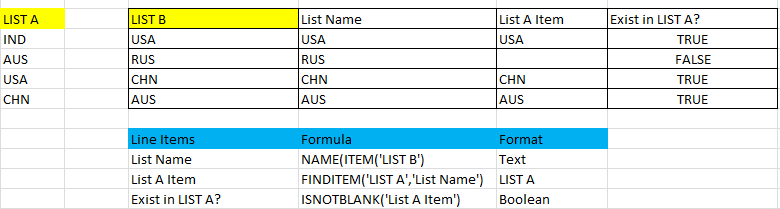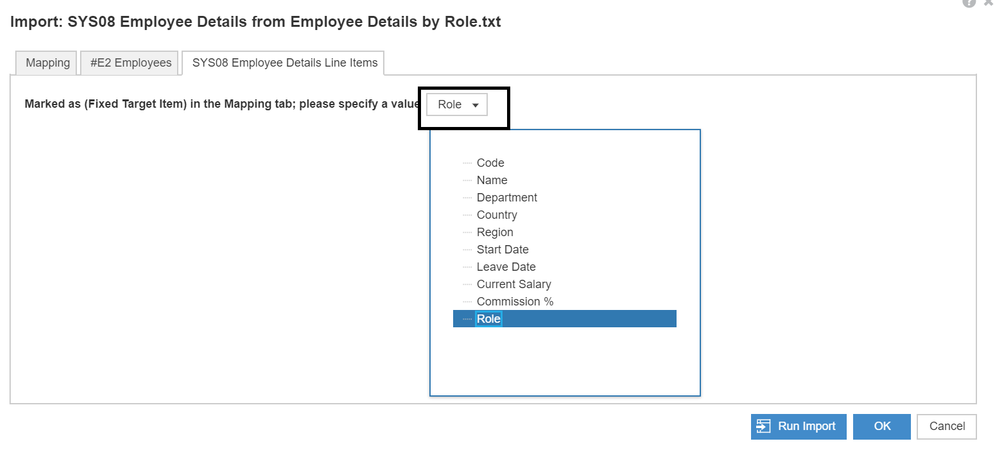# Referring Property values of one List from another ListHi Team,

I am trying to pull the values to my numbered list property from another flat list property.

Here L1 and L2 are my lists and A, B, C are my properties as below.  I need to pull data to property B (text or list formatted) from property C.

 L1 (numbered list) A (list formatted to L2) B

 L2 (flat list) C (list formatted )

Is there any way we could achieve this? Let me know if you need more details on this.

Thanks,

Sandeep

Tagged:

•Hi Sandeep,

Per the best practices, the properties should never reside in the list but the modules which are dimensioned by the respective list. This is per the guidelines of DISCO, per the ideal performance, per the size of the model.

Coming to the question, this can be easily achieved if we have a relation between the 2 lists (L1 and L2) in your case. You will need to only do a lookup or first nonblank based on whether the relation is in the destination list or the source list.

e.g. When we have 2 lists (L1 and L2), L1 has a relation with L2 and hence a property (best practice is that L2 format line item which is dimensioned by L1)Here the link between L1 and L2 is that they have same code and hence I have used the formula 'FINDITEM('L2', CODE(ITEM('L1')))' here for L2 Line item

Now in the module with L2 as dimension, we will have a formula I use the below formula to get the resultThis gets my property to the list L2, which is a numbered list.

We can achieve this also by getting a relation of L1 in the list L2 and then do a lookup

Hope this helps

Thanks

AK

•Thanks @akhil.kohli .

Just a quick question,

Here in my case data, we have in a numbered list are only with a combination of properties.

Can we pull the data to L1 property?

Like I had mentioned before, the only link we have here is, one of the properties in L2 (numbered list ) formatted to L1 list type.

Let me know if you need more details on this.

Thanks,

Sandeep

•Hi Sandeep,

If I understand your questions correctly, you have properties in a numbered list L2 which also has a property of List L1. You need to get these properties in L1 List. Right ?

If this is the case, the above explanation I gave should work. Lets say the property in L2 is Prop1. You need to have this in L1 as well. The formula in this case should be

L1.Prop1 = L2.Prop1[Firstnonblank: L1 List Reference]

Thanks !

AK

•If I understand what you are saying that is the problem with using Combination of Properties, you really don't have a code that you can use.  May I ask why you are using Combination of Properties instead of creating a true code?  When using numbered lists, it is imperative to have a code so you can link other lists to it as stated by Akhil stated.

Thanks,

Rob

•Yeah, I do understand the importance of true code while creating the numbered list.

Here this is my Staging list I have created to load data into the module. This staging list gets deleted after the data load happens. Once I load all the required combination to the numbered list, I have a formula in the property which converts the data into a loadable format.

Here, I need to refer one property from another list (flat list) without creating a separate module or so.

Any help would be really appreciated.

-Sandeep

•Ok, I am going to play devil's advocate here because it is not a good practice to load a into a list (using combination of properties) and do what you need to do, and then delete that list.  It is bad for Hyperblock as well as takes up time in doing the delete and then load again.  A better way of doing this is to understand the data, figure out what really makes the data unique, create a code to make it unique, and then load that.  We have seen many folks loading transactional data as well as the Time period using a combination of properties which over inflates the list which also increases the model opening times.  For example, I found a list of 168k members, but after analyzing the data and understanding what makes it unique and creating that code, the overall list size decreased to only 638 members.  This was accomplished by:

• Creating a unique code for the list
• Creating a transactional module for the transactional data (data) which was dimensionalized by Time and the just created list
• Creating a Properties module of that list to store all properties/meta data.  This is where you would create the link to the other list, either by loading in the code of that list and doing a finditem() to make that "link" or a better way is having the code of the flat list as part of this code.  Then you can simply parse out the codes of the flat lists.  For example, lets say your code is 100_200_300 where 100 is a code for Company, 200 is a code for Region, and 300 is a code for Rep.  Company, Region, and Rep could all be flat lists and now you have links to all of them.

So, is there any way to review the data to see what really makes it unique?

Hope this helps,

Rob